PEAK-Rules/Criteria UserPreferences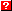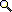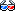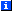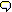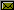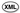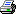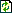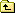Logical Criteria

In order to process arbitrary expression-based rules, PEAK-Rules needs to "understand" the way that conditions logically relate to each other. This document describes the design (and tests the implementation) of its logical criteria management. You do not need to read this unless you are extending or interfacing with this subsystem directly, or just want to understand how this stuff actually works!

The most important ideas here are implication, intersection, and disjunctive normal form. But don't panic if you don't know what those terms mean! They're really quite simple.

Implication means that if one thing is true, then so is another. A implies B if B is always true whenever A is true. It doesn't matter what B is when A is not true, however. It could be true or false, we don't care. Implication is important for prioritizing which rules are "more specific" than others.

Intersection just means that both things have to be true for a condition to be true - it's like the "and" of two conditions. But rather than performing an actual "and", we're creating a new condition that will only be true when the two original conditions would be true.

And finally, disjunctive normal form (DNF) means "an OR of ANDs". For example, this expression is in DNF:

(A and C) or (B and C) or (A and D) or (B and D)

But this equivalent expression is not in DNF:

(A or B) and (C or D)

The criteria used to define generic function methods are likely to look more like this, than they are to be in disjunctive normal form. Therefore, we must convert them in order to implement the Chambers & Chen dispatch algorithm correctly (see Indexing.txt).

We do this using the DisjunctionSet and OrElse classes to represent overall expressions (sets or sequences of "ors"), and the Signature and Conjunction classes to represent sequences or sets of "and"-ed conditions.

Within a Signature, the things that are "and"-ed together are a sequence of Test instances. A Test pairs a "dispatch expression" with a "criterion". For example, this expression:

isinstance(x, Y)

would be represented internally as a Test instance like this:

Test(IsInstance(Local('x')), Class(Y))

Conjunction instances, on the other hand, are used to "and" together criteria that apply to the same dispatch expression. For example, this expression:

isinstance(x, Y) and isinstance(x, Z)

would be represented internally like this:

Test(IsInstance(Local('x')), Conjunction([Class(Y), Class(Z)]))

The rest of this document describes how predicates, signatures, tests, dispatch expressions, and criteria work together to create expressions in disjunctive normal form, and whose implication of other expressions can be determined.

The basic logical functions we will use are implies(), intersect(), disjuncts(), and negate(), all of which are defined in peak.rules.core:

>>> from peak.rules.core import implies, intersect, disjuncts, negate

Boolean Conditions and Logical Operators

The most fundamental conditions are simply True and False. True represents a rule that always applies, while False represents a rule that never applies. Therefore, the result of intersecting True and any other object, always returns that object, while intersecting False with any other object returns False:

>>> intersect(False, False)
False
>>> intersect(False, True)
False
>>> intersect(True, False)
False
>>> intersect(True, True)
True

>>> intersect(object(), True)
<object object at ...>

>>> intersect(True, object())
<object object at ...>

>>> intersect(object(), False)
False

>>> intersect(False, object())
False

Because True means "condition that always applies", everything implies True, but True only implies itself:

>>> implies(object(), True)
True

>>> implies(True, object())
False

>>> implies(True, True)
True

On the other hand, because False means "condition that never applies", False implies everything. (Because if you start from a false premise, you can arrive at any conclusion!):

>>> implies(False, True)
True

>>> implies(False, object())
True

However, no condition other than False can ever imply False, because all other conditions can sometimes apply:

>>> implies(object(), False)
False

>>> implies(True, False)
False

>>> implies(False, False)
True

Notice, by the way, a few important differences between implies() and intersect(). implies() always returns a boolean value, True or False, because it's an immediate answer to the question of, "does the second condition always apply if the first condition applies?"

intersect(), on the other hand, returns a condition that will always be true when the original conditions apply. So, if it returns a boolean value, that's just an indication that the intersection of the two input conditions would always apply or never apply. Also, intersect() is logically symmetrical, in that it doesn't matter what order the arguments are in, whereas the order is critically important for implies().

However, intersect() methods must be order preserving, because the order in which logical "and" operations occur is important. Consider, for example, the condition "y!=0 and z>x/y", in which it would be a bad thing to skip the zero check before the division!

So, as we will see later on, when working with more complex conditions, intersect() methods must ensure that the subparts of the output condition are in the same relative order as they were in the input.

(Also, note that in general, when you intersect two conditions, if one condition implies the other, the result of the intersection is the implying condition. This general rule greatly simplifies the implementation of most intersect operations, since as long as there is an implication relationship defined between conditions, many common cases of intersection can be handled automatically.)

In contrast to both implies() and intersects(), the disjuncts() function takes only a single argument, and returns a list of the "disjuncts" (or-ed-together conditions) of its argument. More precisely, it returns a list of conditions that each imply the original condition. That is, if any of the disjuncts were true, then the original condition would also be true.

Thus, the disjuncts() of an arbitrary object will normally be a list containing just that object:

>>> disjuncts(object())
[<object object at ...>]

>>> disjuncts(True)
[True]

But False is a special case; False has no disjuncts, since no other condition can ever imply False:

>>> disjuncts(False)
[]

As a result, "or"-ing False with other conditions will simply remove the False from the resulting predicate, and conditions that can never be true are not used for indexing or dispatching.

Another special case is tuples containing nested tuples:

>>> disjuncts( (float, (int, str)) )
[(<type 'float'>, <type 'int'>),
(<type 'float'>, <type 'str'>)]

>>> disjuncts( ((int, str), object) )
[(<type 'int'>, <type 'object'>),
(<type 'str'>, <type 'object'>)]

>>> disjuncts( (object, (int, str), float) )
[(<type 'object'>, <type 'int'>, <type 'float'>),
(<type 'object'>, <type 'str'>, <type 'float'>)]

>>> disjuncts( ((int, str), (int, str)) )
[(<type 'int'>, <type 'int'>),
(<type 'str'>, <type 'int'>),
(<type 'int'>, <type 'str'>),
(<type 'str'>, <type 'str'>)]

This lets you avoid writing lots of decorators for the cases where you want more than one type (or istype() instance) to match in a given argument position. (As you can see, it's equivalent to specifying all the individual combinations of specified types.)

Finally, the negate() function inverts the truth of a condition, e.g.:

>>> negate(True)
False

>>> negate(False)
True

Of course, it also applies to criteria other than pure boolean values, as we'll see in the upcoming sections.

"Criterion" Objects

A criterion object describes a set of possible values for a dispatch expression. There are several criterion types supplied with PEAK-Rules, but you can also add your own, as long as they can be tested for implication with implies(), and intersected with intersect(). (And if they represent an "or" of sub-criteria, they should be able to provide their list of disjuncts(). They'll also need to be indexable, but more on that later in other documents!)

"And"-ed Criteria

Sometimes, more than one criterion is applied to the same dispatch expression. For example in the expression x is not y and x is not z, two criteria are being applied to the identity of x. To represent this, we need a way to represent a set of "and-ed" criteria. peak.rules.criteria provides a base class for this, called Conjunction:

>>> from peak.rules.criteria import Conjunction

This class is a subclass of frozenset, but has a few additional features. First, a Conjunction never contains redundant (implied) items. For example, the conjunction of the classes object and int is int, because int already implies object:

>>> Conjunction([int, object])
<type 'int'>

>>> Conjunction([object, int])
<type 'int'>

Notice also that instead of getting back a set with one member, we got back the item that would have been in the set. This helps to simplify the expression structure. As a further simplification, creating an empty conjunction returns True, because "no conditions required" is the same as "always true":

>>> Conjunction([])
True

A conjunction implies a condition, if any condition in the conjunction implies the other condition:

>>> implies(Conjunction([str, int]), str)
True
>>> implies(Conjunction([str, int]), int)
True
>>> implies(Conjunction([str, int]), object)
True
>>> implies(Conjunction([str, int]), float)
False

A condition implies a conjunction, however, only if the condition implies every part of the conjunction:

>>> class a: pass
>>> class b: pass
>>> class c(a,b): pass
>>> class d(a, int): pass

>>> implies(c, Conjunction([a, b]))
True
>>> implies(a, Conjunction([a, b]))
False

>>> implies(Conjunction([c,d]), Conjunction([a, int]))
True
>>> implies(Conjunction([c, int]), Conjunction([a, int]))
True
>>> implies(Conjunction([a, int]), Conjunction([c, int]))
False

(By the way, on a more sophisticated level of reasoning, you could say that Conjunction([str, int]) should have equalled False above, since there's no way for an object to be both an int and a str at the same time. But that would be an excursion into semantics and outside the bounds of what PEAK-Rules can "reason" about using only logical implication as defined by the implies() generic function.)

Conjunction objects can be intersected with one another, or with additional conditions, and the result is another Conjunction of the same type as the leftmost set. So, if we use subclasses of our own, the result of intersecting them will be a conjunction of the correct subclass:

>>> class MySet(Conjunction): pass

>>> type(intersect(MySet([int, str]), float))
<class 'MySet'>

>>> intersect(MySet([int, str]), float) == MySet([int, str, float])
True

>>> intersect(float, MySet([int, str])) == MySet([float, int, str])
True

>>> intersect(MySet([d, c]), MySet([int, str])) == MySet([d,c,str])
True

If you want to ensure that all items in a set are of appropriate type or value, you can override __init__ to do the checking, and raise an appropriate error. PEAK-Rules does this for its specialized conjunction classes, but uses if __debug__: and assert statements to avoid the extra overhead when run with python -O. You may wish to do the same for your subclasses.

"Or"-ed Criteria

The DisjunctionSet and OrElse classes are used to represent sets and sequences of "or"-ed criteria:

>>> from peak.rules.criteria import DisjunctionSet, OrElse

Both types automatically exclude redundant (i.e. more-specific) criteria, and can never contain less than 2 entries. For example, "or"-ing object and int always returns object, because object is implied by int:

>>> DisjunctionSet([int, object])
<type 'object'>

>>> DisjunctionSet([object, int])
<type 'object'>

>>> OrElse([int, object])
<type 'object'>

>>> OrElse([object, int])
<type 'object'>

Notice that instead of getting back a set or sequence with one member, we got back the item that would have been in the set. This helps to simplify the expression structure. As a further simplification, creating an empty disjunction returns False, because "no conditions are sufficient" is the same as "always false":

>>> DisjunctionSet([])
False

>>> OrElse([])
False

In addition to eliminating redundancy, disjunction sets also flatten any nested disjunctions:

>>> DisjunctionSet([DisjunctionSet([1, 2]), DisjunctionSet([3, 4])])
DisjunctionSet([1, 2, 3, 4])

This is because it uses the disjuncts() generic function to determine whether any of the items it was given are "or"-ed conditions of some kind. And the disjuncts() of a DisjunctionSet are a list of its contents:

>>> disjuncts(DisjunctionSet([1, 2, 3, 4]))
[1, 2, 3, 4]

But OrElse sequences do not do this flattening, in order to avoid imposing an arbitrary sequence on their contents:

>>> OrElse([DisjunctionSet([1, 2]), DisjunctionSet([3, 4])])
OrElse([DisjunctionSet([1, 2]), DisjunctionSet([3, 4])])

(The disjuncts() of an OrElse are much more complicated, as the disjuncts of a Python expression like "a or b or c" reduce to "a", "(not a) and b", and "(not a and not b) and c"! We'll talk more about this later, in the section on Predicates below.)

A disjunction only implies a condition if all conditions in the disjunction imply the other condition:

>>> implies(DisjunctionSet([str, int]), str)
False
>>> implies(DisjunctionSet([str, int]), int)
False
>>> implies(DisjunctionSet([str, int]), float)
False
>>> implies(DisjunctionSet([str, int]), object)
True

>>> implies(OrElse([str, int]), str)
False
>>> implies(OrElse([str, int]), int)
False
>>> implies(OrElse([str, int]), float)
False
>>> implies(OrElse([str, int]), object)
True

A condition implies a disjunction, however, if the condition implies any part of the disjunction:

>>> class a: pass
>>> class b: pass
>>> class c(a,b): pass
>>> class d(a, int): pass

>>> implies(c, DisjunctionSet([a, b]))
True
>>> implies(a, DisjunctionSet([a, b]))
True
>>> implies(a, DisjunctionSet([int, str]))
False
>>> implies(DisjunctionSet([c,d]), DisjunctionSet([a, int]))
True
>>> implies(DisjunctionSet([c,int]), DisjunctionSet([a, int]))
True

>>> implies(DisjunctionSet([c, int]), True)
True
>>> implies(False, DisjunctionSet([c, int]))
True

>>> implies(c, OrElse([a, b]))
True
>>> implies(a, OrElse([a, b]))
True
>>> implies(a, OrElse([int, str]))
False
>>> implies(OrElse([c,d]), OrElse([a, int]))
True
>>> implies(OrElse([c,int]), OrElse([a, int]))
True

>>> implies(OrElse([c, int]), True)
True
>>> implies(False, OrElse([c, int]))
True

The intersection of a disjunction and any other object is a disjunction containing the intersection of that object with the original disjunctions' contents. In other words:

>>> int_or_str = DisjunctionSet([int, str])
>>> long_or_float = DisjunctionSet([long, float])

>>> intersect(int_or_str, float) == DisjunctionSet([
...     Conjunction([int, float]), Conjunction([str, float])
... ])
True

>>> intersect(long, int_or_str) == DisjunctionSet([
...     Conjunction([long, int]), Conjunction([long, str])
... ])
True

>>> intersect(int_or_str, long_or_float) == DisjunctionSet([
...     Conjunction([int,long]), Conjunction([int, float]),
...     Conjunction([str,long]), Conjunction([str, float]),
... ])
True

>>> intersect(int_or_str, Conjunction([long, float])) == \
...     DisjunctionSet(
...         [Conjunction([int, long, float]),
...          Conjunction([str, long, float])]
... )
True

>>> intersect(Conjunction([int, str]), long_or_float) == \
...     DisjunctionSet(
...         [Conjunction([int, str, long]), Conjunction([int, str, float])]
...     )
True

As you can see, this is the heart of the process that allows expressions like (A or B) and (C or D) to be transformed into their disjunctive normal form (i.e. (A and C) or (A and D) or (B and C) or (B and D)).

(In other words, by using Disjunction() as an "or" operator and intersect() as the "and" operator, we always end up with a DNF result!)

Object Identity

The IsObject criterion type represents the set of objects which either are -- or are not -- one specific object instance. IsObject(x) (or IsObject(x, True)) represents the set of objects y for which the y is x condition would be true. Conversely, IsObject(x, False) represents the set of objects y for whom y is not x:

>>> from peak.rules.criteria import IsObject, Conjunction

>>> o = object()
>>> is_o = IsObject(o)
>>> is_not_o = IsObject(o, False)

>>> is_o
IsObject(<object object at ...>, True)

>>> is_not_o
IsObject(<object object at ...>, False)

>>> is_not_o == negate(is_o)
True

>>> is_o == negate(is_not_o)
True

The intersection of two different is identities is False, since an object cannot be both itself and another object:

>>> intersect(is_o, IsObject("foo"))
False

>>> implies(is_o, IsObject("foo"))
False

Similarly, an object can't be both itself, and not itself:

>>> intersect(is_o, is_not_o)
False

>>> intersect(is_not_o, is_o)
False

>>> implies(is_o, is_not_o)
False

But it can be itself and itself:

>>> intersect(is_o, is_o) == is_o
True

>>> implies(is_o, is_o)
True

Or not itself and not itself:

>>> intersect(is_not_o, is_not_o) == is_not_o
True

>>> implies(is_not_o, is_not_o)
True

And an object can be itself, while not being something else:

>>> intersect(is_o, IsObject("foo", False)) == is_o
True

>>> intersect(IsObject("foo", False), is_o) == is_o
True

>>> implies(is_o, IsObject("foo", False))
True

But just because an object is not something, doesn't mean it's something else:

>>> implies(is_not_o, IsObject("foo"))
False

And the intersection of multiple is not conditions produces a Conjunction:

>>> not_foo = IsObject("foo", False)
>>> not_bar = IsObject("bar", False)
>>> not_foobar = intersect(not_foo, not_bar)
>>> not_foobar
Conjunction([IsObject('foo', False), IsObject('bar', False)])

Which of course then implies each of the individual "not" conditions:

>>> implies(not_foobar, not_bar)
True
>>> implies(not_foobar, not_foo)
True

But not their opposites:

>>> implies(not_foobar, IsObject("bar"))
False

Oh, and an is condition implies any Conjunction that don't contain its opposite:

>>> implies(is_o, not_foobar)
True

But not the other way around:

>>> implies(not_foobar, is_o)
False

Finally, negating a Conjunction of is-nots returns a disjunction of true IsObject tests, and vice versa:

>>> negate(not_foobar)
DisjunctionSet([IsObject('foo', True), IsObject('bar', True)])

>>> negate(DisjunctionSet([IsObject('foo'), IsObject('bar')]))
Conjunction([IsObject('foo', False), IsObject('bar', False)])

Values and Ranges

Value objects are used to represent == and != comparisons. Value(x) represents ==x and Value(x, False) represents !=x.

A Value implies another Value if the two are identical:

>>> from peak.rules.criteria import Value, Range, Min, Max

>>> implies(Value(27), Value(42))
False
>>> implies(Value(27, False), Value(42))
False
>>> implies(Value(27), Value(27))
True
>>> implies(Value(99), Value(99, False))
False
>>> implies(Value(99, False), Value(99, False))
True

Or, if they have different target values, but the first is an == comparison, and the second is a != comparison:

>>> implies(Value(27), Value(99, False))
True

>>> intersect(Value(27), Value(99, False))
Value(27, True)

The negation of a Value is of course another Value of the same target but the reverse operator:

>>> negate(Value(27))
Value(27, False)

>>> negate(Value(99, False))
Value(99, True)

The intersection of two different == values, or a != and == of the same value, is False (i.e., no possible match:

>>> intersect(Value(27), Value(42))
False
>>> intersect(Value(27), Value(27, False))
False

But the intersection of two different != values produces a disjunction of three Range() objects:

>>> one_two = intersect(Value(1, False), Value(2, False))

>>> one_two == DisjunctionSet([
...     Range((Min, -1), (1, -1)),
...     Range((1, 1), (2, -1)),
...     Range((2, 1), (Max, 1))
... ])
True

>>> intersect(one_two, Value(3,False)) == DisjunctionSet([
...     Range((Min, -1), (1, -1)),
...     Range((1, 1), (2, -1)),
...     Range((2, 1), (3, -1)),
...     Range((3, 1), (Max, 1))
... ])
True

The Range() criterion type represents an inequality such as lo < x < hi or x >= lo. The lows and highs given have to be a 2-tuple, consisting of a value and a "direction". The direction is an integer (either -1 or 1) that indicates whether the edge is on the low or high side of the target value. Thus, a tuple (27, -1) means "the low edge of 27", while (99, 1) means "the high edge of 99". In this way, any simple inequality or range can be represented by a pair of edges.

Thus, the intersection of two different != values produces a disjunction of three Range() objects, representing the intervals that "surround" the original != values:

>>> from peak.rules.criteria import Range

>>> intersect(Value(27, False), Value(42, False)) == DisjunctionSet([
...     Range(hi=(27, -1)),     # below Min ... below 27
...     Range((27,1), (42,-1)), # above  27 ... below 42
...     Range(lo=(42, 1)),      # above  42 ... above Max
... ])
True

Notice that if we omit the hi or lo, end of the range, it's replaced with "below Min" or "above Max", as appropriate. (The Min and Max values are special objects that compare below or above any other object.)

When creating range and value objects, it can be useful to use the Inequality constructor, which takes a comparison operator and a value:

>>> from peak.rules.criteria import Inequality

>>> Inequality('>=', 27)    # >=27 : below 27 ... above Max
Range((27, -1), (Max, 1))

>>> negate(Inequality('<', 27))
Range((27, -1), (Max, 1))

>>> Inequality('>', 27)     # > 27 : above 27 ... above Max
Range((27, 1), (Max, 1))

>>> Inequality('<', 99)     # < 99 : below Min ... below 99
Range((Min, -1), (99, -1))

>>> Inequality('<=',99)     # <=99 : below Min ... above 99
Range((Min, -1), (99, 1))

>>> negate(Inequality('>', 99))
Range((Min, -1), (99, 1))

>>> Inequality('==', 66)
Value(66, True)

>>> Inequality('!=', 77)
Value(77, False)

Intersecting two ranges (or a range and an == value) produces a smaller range or value, or False if there is no overlap:

>>> intersect(Inequality('<', 27), Inequality(">",19))
Range((19, 1), (27, -1))

>>> intersect(Inequality('>=', 27), Inequality("<=",19))
False

>>> intersect(Value(27), Inequality('>=', 27))
Value(27, True)
>>> intersect(Inequality('<=', 27), Value(27))
Value(27, True)

>>> intersect(Value(27), Inequality('<',27))
False
>>> intersect(Inequality('>',27), Value(27))
False

Last, but not least, a range (or value) implies another range or value if it lies entirely within it:

>>> implies(Range((42,-1), (42,1)), Value(42))
True

>>> implies(Range((27,-1), (42,1)), Range((15,1),(99,-1)))
True

>>> implies(Range((27,-1), (42,1)), Value(99, False))
True

But not if it overlaps or lies outside of it:

>>> implies(Range((15,-1),(42,1)), Range((15,1),(99,-1)))
False

>>> implies(Range((27,-1), (42,1)), Value(99))
False

Class/Type Tests

Class objects represent issubclass() or isinstance() sets. Class(x) is a instance/subclass match, while Class(x, False) is a non-match. Implication, negation, and intersection are defined accordingly:

>>> from peak.rules.criteria import Class

>>> implies(Class(int), Class(object))
True
>>> implies(Class(object, False), Class(int, False))
True

>>> negate(Class(int))
Class(<type 'int'>, False)

>>> negate(Class(object, False))
Class(<type 'object'>, True)

>>> implies(Class(int), Class(str))
False
>>> implies(Class(object), Class(int, False))
False
>>> implies(Class(object), Class(int))
False
>>> implies(Class(int), Class(int))
True

>>> intersect(Class(int), Class(object))
Class(<type 'int'>, True)

>>> intersect(Class(object), Class(int))
Class(<type 'int'>, True)

The intersection of two or more unrelated Class criteria is represented by a Conjunction:

>>> from peak.rules.criteria import Conjunction

>>> intersect(Class(int, False), Class(str, False)) == Conjunction(
...     [Class(int, False), Class(str, False)]
... )
True

Exact-Type and Type-Exclusion Tests

Exact type tests are expressed using istype(x), and type exclusion tests are represented as istype(x, False):

>>> from peak.rules import istype

>>> negate(istype(int))
istype(<type 'int'>, False)

>>> negate(istype(object, False))
istype(<type 'object'>, True)

One istype() test implies another only if they're equal:

>>> implies(istype(int), istype(int))
True

>>> implies(istype(int, False), istype(int, False))
True

>>> implies(istype(int, False), istype(int))
False

Or if the first is an exact match, and the second is an exclusion test for a different type:

>>> implies(istype(int), istype(str, False))
True

Thus, the intersection of two istype() tests will be either one of the input tests, or False (meaning no overlap):

>>> intersect(istype(int), istype(int))
istype(<type 'int'>, True)

>>> intersect(istype(int), istype(str, False))
istype(<type 'int'>, True)

>>> intersect(istype(int, False), istype(int, False))
istype(<type 'int'>, False)

>>> intersect(istype(int), istype(str))
False

Unless both are exclusion tests on different types, in which case their intersection is a Conjunction of the two:

>>> intersect(istype(str, False), istype(int, False)) == Conjunction([
...     istype(int, False), istype(str, False)
... ])
True

An istype(x) implies Class(y) only if x is y or a subtype thereof:

>>> implies(istype(int), Class(str))
False

>>> implies(istype(int), Class(object))
True

And it implies Class(y, False) only if x is not y or a subtype thereof:

>>> implies(istype(int), Class(str, False))
True

>>> implies(istype(int), Class(object, False))
False

But istype(x, False) implies nothing about any Class test, since it refers to exactly one type, while the Class may refer to infinitely many types:

>>> implies(istype(int, False), Class(int, False))
False

>>> implies(istype(int, False), Class(object))
False

Meanwhile, Class(x) tests can only imply istype(y, False), where y is a superclass of x:

>>> implies(Class(int), istype(int))
False

>>> implies(Class(int), istype(object))
False

>>> implies(Class(int), istype(object, False))
True

And Class(x, False) cannot imply anything about any istype() test, whether true or false:

>>> implies(Class(int, False), istype(int))
False

>>> implies(Class(int, False), istype(int, False))
False

When Class() is intersected with an exact type test, the result is either the exact type test, or False:

>>> intersect(Class(int), istype(int))
istype(<type 'int'>, True)

>>> intersect(istype(int), Class(int))
istype(<type 'int'>, True)

>>> intersect(Class(int), istype(object))
False

>>> intersect(istype(object), Class(int))
False

>>> intersect(Class(int, False), istype(object))
istype(<type 'object'>, True)

>>> intersect(istype(object), Class(int, False))
istype(<type 'object'>, True)

But when it's intersected with a type exclusion test, the result is a Conjunction:

>>> intersect(istype(int, False), Class(str)) == Conjunction([
...     istype(int, False), Class(str, True)
... ])
True

>>> s = intersect(Class(str), istype(int, False))
>>> s == Conjunction([istype(int, False), Class(str, True)])
True

>>> intersect(s, istype(int))
False

>>> intersect(s, istype(int, False)) == s
True

>>> intersect(s, istype(str))
istype(<type 'str'>, True)

Tests and Signatures

Test Objects

A Test is the combination of a "dispatch expression" and a criterion to be applied to it:

>>> from peak.rules.criteria import Test
>>> x_isa_int = Test("x", Class(int))

(Note that although these examples use strings, actual dispatch expressions will be AST-like structures.)

Creating a test with disjunct criteria actually returns a set of tests:

>>> Test("x", DisjunctionSet([int, str])) == \
...           DisjunctionSet([Test('x', int), Test('x', str)])
True

So the disjuncts() of a test will always just be the test itself:

>>> disjuncts(x_isa_int)
[Test('x', Class(<type 'int'>, True))]

Negating a test usually just negates its criterion, leaving the expression intact:

>>> negate(x_isa_int)
Test('x', Class(<type 'int'>, False))

But if the test criterion is a conjunction or range, negating it can produce a disjunction of tests:

>>> negate(
...     Test('x',
...         Conjunction([IsObject('foo',False), IsObject('bar',False)])
...     )
... ) == DisjunctionSet(
...     [Test('x', IsObject('foo', True)),
...      Test('x', IsObject('bar', True))])
True

Intersecting two tests for the same dispatch expression returns a test whose criterion is the intersection of the original tests' criteria:

>>> intersect(x_isa_int, Test("x", Class(str))) == Test(
...     'x', Conjunction([Class(int), Class(str)])
... )
True

And similarly, a test only implies another test if they have equal dispatch expressions, and the second test's criterion is implied by the first's:

>>> implies(x_isa_int, Test("x", Class(str)))
False
>>> implies(x_isa_int, Test("x", Class(object)))
True
>>> implies(x_isa_int, Test("y", Class(int)))
False

But the intersection of two tests with different dispatch expressions produces a Signature object:

>>> y_isa_str = Test("y", Class(str))
>>> x_int_y_str = intersect(x_isa_int, y_isa_str)
>>> x_int_y_str
Signature([Test('x', Class(...int...)), Test('y', Class(...str...))])

Signature Objects

Signature objects are similar to Conjunction objects, except for three important differences.

First, signatures are sequences, not sets. They preserve the ordering they were created with:

>>> intersect(x_isa_int, y_isa_str)
Signature([Test('x', Class(...int...)), Test('y', Class(...str...))])

>>> intersect(y_isa_str, x_isa_int)
Signature([Test('y', Class(...str...)), Test('x', Class(...int...))])

and their negations preserve the order as well (using OrElse instances):

>>> negate(intersect(x_isa_int, y_isa_str))
OrElse([Test('x', Class(...int..., False)),
Test('y', Class(...str..., False))])

>>> negate(intersect(y_isa_str, x_isa_int))
OrElse([Test('y', Class(...str..., False)),
Test('x', Class(...int..., False))])

Second, signatures can only contain Test instances, and they automatically intersect() any tests that apply to the same dispatch signatures:

>>> from peak.rules.criteria import Signature

>>> intersect(x_int_y_str, Test("y", Class(float))) == Signature([
...     Test('x', Class(int)),
...     Test('y', Conjunction([Class(str), Class(float)]))
... ])
True

>>> intersect(x_int_y_str, Test("x", Class(float))) == Signature([
...     Test('x', Conjunction([Class(int), Class(float)])),
...     Test('y', Class(str))
... ])
True

>>> intersect(Test("x", Class(float)), x_int_y_str) == Signature([
...     Test('x', Conjunction([Class(int), Class(float)])),
...     Test('y', Class(str))
... ])
True

But, as with conjunctions, you can't create a signature with less than two items in it:

>>> Signature([Test("x",1)])
Test('x', 1)

>>> Signature([True])
True

>>> Signature([False])
False

>>> Signature([])
True

Predicates

Now that we've got all the basic pieces in place, we can now operationally define predicates for the Chambers & Chen dispatch algorithm.

Specifically, a predicate can be any of the following:

• True (meaning a condition that always applies)
• False (meaning a condition that never applies)
• A Test or Signature instance
• A DisjunctionSet or OrElse containing two or more Test or Signature instances

In each case, invoking disjuncts() on the object in question will return a list of objects suitable for constructing dispatch "cases" -- i.e., sets of simple "and-ed" criteria that can easily be indexed.

The tests_for() function can then be used to yield the component tests of each case signature. When called on a Test, it yields the given test:

>>> from peak.rules.criteria import tests_for

>>> list(tests_for(Test('y',42)))
[Test('y', 42)]

But called on a Signature, it yields the tests contained within:

>>> list(tests_for(x_int_y_str))
[Test('x', Class(...int...)), Test('y', Class(...str...))]

And called on True, it yields nothing:

>>> list(tests_for(True))
[]

tests_for(False), however, is undefined, because False cannot be represented as a conjunction of tests. False is still a valid predicate, of course, because it represents an empty disjunction.

In normal predicate processing, one loops over the disjuncts() of a predicate, and only then uses tests_for() to inspect the individual items. But since disjuncts(False) is an empty list, it should never be necessary to invoke tests_for(False).

There is an important distinction, however, in how disjuncts() works on OrElse objects, compared to all other kinds of predicates. disjuncts() is used to obtain the unordered disjunctions of a logical condition, but OrElse is ordered, because it represents a series of applications of the Python "or" operator.

In Python, a condition on the right-hand side of an "or" operator is not tested unless the condition on the left is false. PEAK-Rules, however, tests the disjuncts() of a predicate independently. Thus, in order to properly translate "or" conditions in a predicate, the disjuncts() of an OrElse must include additional and-ed conditions to force them to be tested in order.

Specifically, the disjuncts() of OrElse([a, b, c]) will be:

• a,
• intersect(negate(a), b), and
• intersect(intersect(negate(a), negate(b)), c)!

This expansion ensures that b will never be tested unless a is false, and c will never be tested unless a and b are both false, just like in a regular Python expression. Observe:

>>> DisjunctionSet([OrElse([Class(a), Class(b)])]) == DisjunctionSet([
...     Class(a, True),
...     Conjunction([Class(a, False), Class(b, True)])
... ])
True

Also, because OrElse objects don't expand their contents' disjuncts at creation time, they must be expanded as part of the disjuncts() operation:

>>> a_or_b = DisjunctionSet([Class(a), Class(b)])

>>> try:
...     set = set
... except NameError:
...     from sets import Set as set     # 2.3, ugh

>>> set(disjuncts(OrElse([istype(int), a_or_b]))) == set([
...     istype(int),
...     Conjunction([istype(int, False), Class(b)]),
...     Conjunction([istype(int, False), Class(a)])
... ])
True

This delayed expansion "preserves the unorderedness" of the contents, by not forcing them to be evaluated in any specific sequence, apart from the requirements imposed by their position within the OrElse.

We'll do one more test, to show that the disjuncts of the negated portions of the OrElse are also expanded:

>>> a_and_b = Conjunction([Class(a), Class(b)])
>>> not_a = Class(a, False)
>>> not_b = Class(b, False)
>>> int_or_str = DisjunctionSet([Class(int), Class(str)])

>>> set(disjuncts(OrElse([a_and_b, int_or_str]))) == set([
...     a_and_b, Conjunction([not_a, Class(int)]), Conjunction([not_a, Class(str)]),
...              Conjunction([not_b, Class(int)]), Conjunction([not_b, Class(str)])
... ])
True

per this expansion logic (using | for "symmetric or"):

(a and b) or (int|str) => (a and b) | not (a and b) and (int|str)

not (a and b) and (int|str) => (not a | not b) and (int|str)

(not a | not b) and (int|str) => (
(not a and int) | (not a and str) | (not b and int) | (not b and str)
)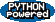EditText of this page (last modified 2011-08-31 21:11:41) FindPage by browsing, title search , text search or an index Or try one of these actions: AttachFile, DeletePage, LikePages, LocalSiteMap, SpellCheck